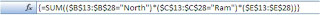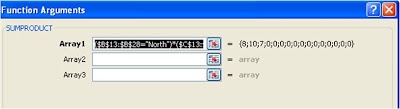## Friday, November 6, 2009

### Excel Formula : Sumif Multiple Criteria

This is in continuation of my earlier post on Countif Multiple Criteria The same logic can be extended to calculate sum based on multiple criteria.

In case you have Excel 2007, you can use built in function Sumifs . This will let you add multiple conditions.In case of Excel 2003 or earlier version using Pivot table will get you the results easily but if You don't have liberty to use Pivot, then you will need formula trick. Consider that you have a data table like this and you need to know what is sales in "North" region by sales man "Ram".

Following sumproduct formula will do the calculation for you.

=SUMPRODUCT((\$B\$13:\$B\$28="North")*(\$C\$13:\$C\$28="Ram")*(\$E\$13:\$E\$28))

Another way is to write an array formula.
=SUM((\$B\$13:\$B\$28="North")*(\$C\$13:\$C\$28="Ram")*(\$E\$13:\$E\$28))
However this will need to be confirmed with CTRL+Shift+Enter (CSE)

After CSE formula will show {} in formula bar. This will covert it to an array formula. The formula will look like following picture after CSE in formula barI suggest you to use Sumproduct formula as you may find it difficult to enter array formula.

If you see the dialog for sumproduct formula entry you will understand the reasons behind this recommendation. Actually sumproduct is a workaround to enter array formula.If you see above dialog screen , you will notice

1. When you enter ranges, you are actually entering array's.
2. This formula is entered in single array only to get the results.

If you try to use sumproduct as normal formula to calculate sum based on multiple conditions , you will not get the results.

Actually multiple condistional sum can be calculated using arrays only and sumproduct allows you to enter array's easily and you can over come the difficulty of entering array formula.

1.2.